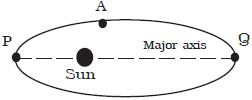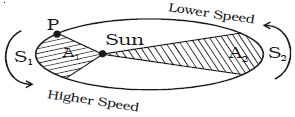# Kepler’s Laws of Planetary Motion

Kepler’s laws of planetary motion

(i) The law of orbits

Each planet moves in an elliptical orbit with the Sun at one focus. A is a planet revolving round the Sun. The position P of the planet where it is very close to the Sun is known as perigee and the position Q of the planet where it is farthest from the Sun is known as apogee.(ii) The law of areas

The line joining the Sun and the planet (Le radius vector) sweeps out equal areas in equal interval of times.

The orbit of the planet around the Sun is as shown in Fig 2. The areas A1 and A2 are swept by the radius vector in equal times. The planet covers unequal distances S1 and S2 in equal time. This is due to the variable speed of the planet. When the planet is closest to the Sun. it covers greater distance in a given time. Hence, the speed is maximum at the closest position. When the planet is far away from the Sun, it covers lesser distance in the same time. Hence the speed is minimum at the farthest position.